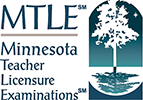## Test Framework Worksheet

Check this box if the course listed above covers the content described by this objective
Check this box if the course listed above covers the content described by this objective
Check this box if the course listed above covers the content described by this objective
Check this box if the course listed above covers the content described by this objective
Check this box if the course listed above covers the content described by this objective
Check this box if the course listed above covers the content described by this objective
Check this box if the course listed above covers the content described by this objective
Check this box if the course listed above covers the content described by this objective
Check this box if the course listed above covers the content described by this objective
Check this box if the course listed above covers the content described by this objective
Check this box if the course listed above covers the content described by this objective
Check this box if the course listed above covers the content described by this objective
Check this box if the course listed above covers the content described by this objective
Check this box if the course listed above covers the content described by this objective
Check this box if the course listed above covers the content described by this objective
This worksheet allows users to map the test framework to the curriculum by entering up to five courses in the course name fields, and checking the boxes if the content of the course covers the information described by the test objectives in the rows below.
Subarea Objective Number Objective Description
Subtest 1: Test Code 054
Subarea I. Number Sense
• Multiple-Choice Questions: 21
• Test Weight: 43%
0001 Understand numbers and number systems.
0002 Understand number theory and operations on numbers.
0003 Apply principles of problem solving.
Subarea II. Functions, Algebra, and Calculus
• Multiple-Choice Questions: 29
• Test Weight: 57%
0004 Understand the properties and representations of functions.
0005 Apply algebraic techniques.
0006 Analyze linear and nonlinear functions and their applications.
0007 Apply knowledge of trigonometry and calculus.
Subtest 2: Test Code 055
Subarea I. Measurement and Geometry
• Multiple-Choice Questions: 19
• Test Weight: 38%
0008 Apply principles of measurement and geometry to solve problems.
0009 Analyze figures and shapes in two and three dimensions.
0010 Analyze figures and shapes using coordinate and transformational geometry.
Subarea II. Data, Probability, and Discrete Math
• Multiple-Choice Questions: 19
• Test Weight: 37%
0011 Apply knowledge of the collection, presentation, and analysis of data.
0012 Understand the principles of probability.
0013 Understand the processes and applications of discrete mathematics.
Subarea III. Mathematical Processes
• Multiple-Choice Questions: 12
• Test Weight: 25%
0014 Understand mathematical connections and communication.
0015 Understand the content and methods for developing students' content-area reading skills to support their reading and learning in mathematics.

Print this worksheet to save for your records, or to fill out offline: